Geometry 9-4 Compositions of Isometries
starstarstarstarstarstarstarstarstarstar
by Matthew Richardson
| 24 Questions
Note from the author:
A complete formative lesson with embedded slideshow, mini lecture screencasts, checks for understanding, practice items, mixed review, and reflection. I create these assignments to supplement each lesson of Pearson's Common Core Edition Algebra 1, Algebra 2, and Geometry courses. See also mathquest.net and twitter.com/mathquestEDU.
The outlined content above was added from outside of Formative.
The outlined content above was added from outside of Formative.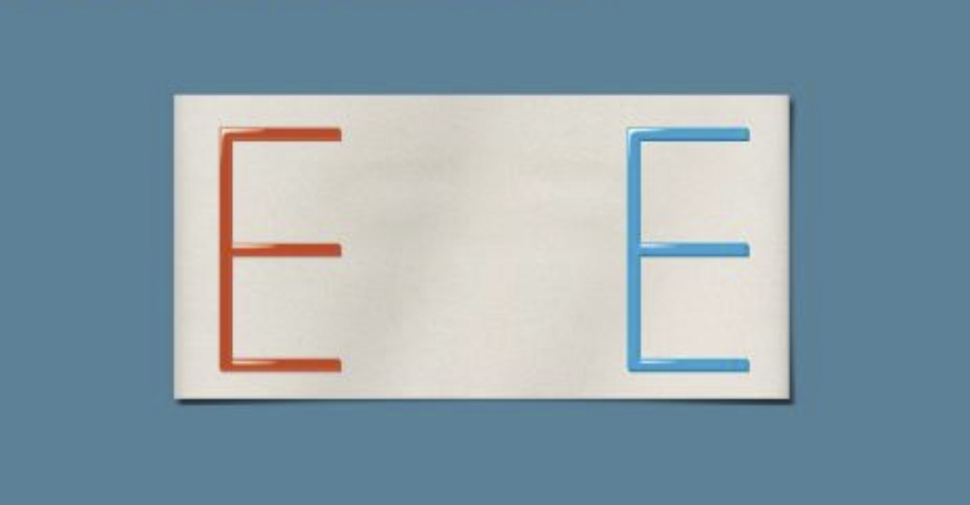1
2
1
10 pts
Solve It! The blue E is a horizontal translation of the red E. How can you use two reflections, one right after the other, to move the red E to the position of the blue E?
2
10 pts
Solve It! Draw the two lines of reflection you used on the image on the canvas.The outlined content above was added from outside of Formative.
3
10 pts
Problem 1 Got It? Lines l and m are copied from Problem 1. Draw J between l and m. Draw the image of the following composition of reflections in blue or green.

4
10 pts
Problem 1 Got It? What is the distance of the resulting translation in the previous item?
5
10 pts
Problem 1 Got It? Reasoning: Use the results of the previous items and Problem 1. Make a conjecture about the distance of any translation that is the result of a composition of reflections across two parallel lines.The outlined content above was added from outside of Formative.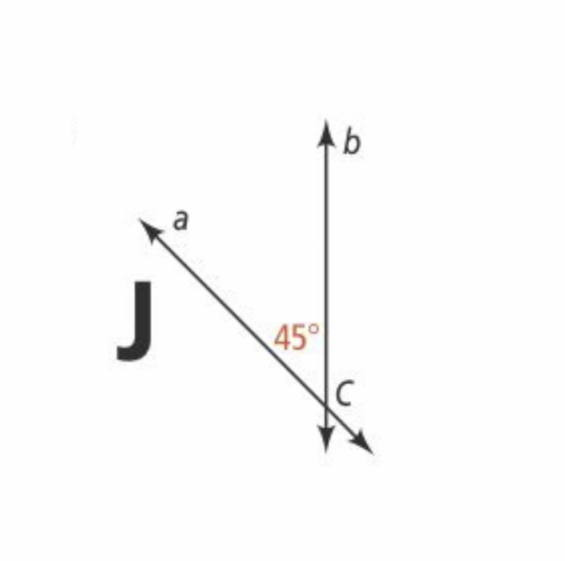6
7
8
9
6
10 pts
Problem 2 Got It? Draw the following composition of reflections on the canvas.
7
10 pts
Problem 2 Got It? What is the center for the resulting rotation?
a
b
C
8
10 pts
Problem 2 Got It? What is the angle of rotation for the resulting rotation?
45° clockwise
90° clockwise
180° clockwise
9
10 pts
Problem 2 Got It? Reasoning: Use the results of the previous items and Problem 2. Make a conjecture about the center of rotation and the angle of rotation for any rotation that is the result of any composition of reflections across two intersecting lines.The outlined content above was added from outside of Formative.
10
10 pts
Problem 3 Got It? Graph ΔTEX in black. Graph the image ΔT'E'X' for the glide reflection in green or blue. Be sure to include relevant graph detail.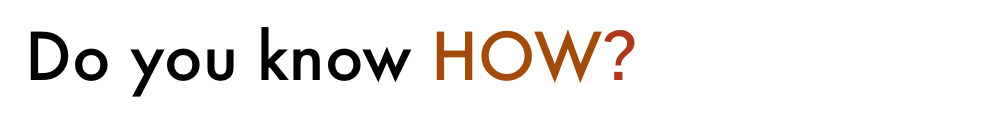11
11
20 pts
For each diagram, sketch the image of Z reflected across line a, then across line b. Use green or blue.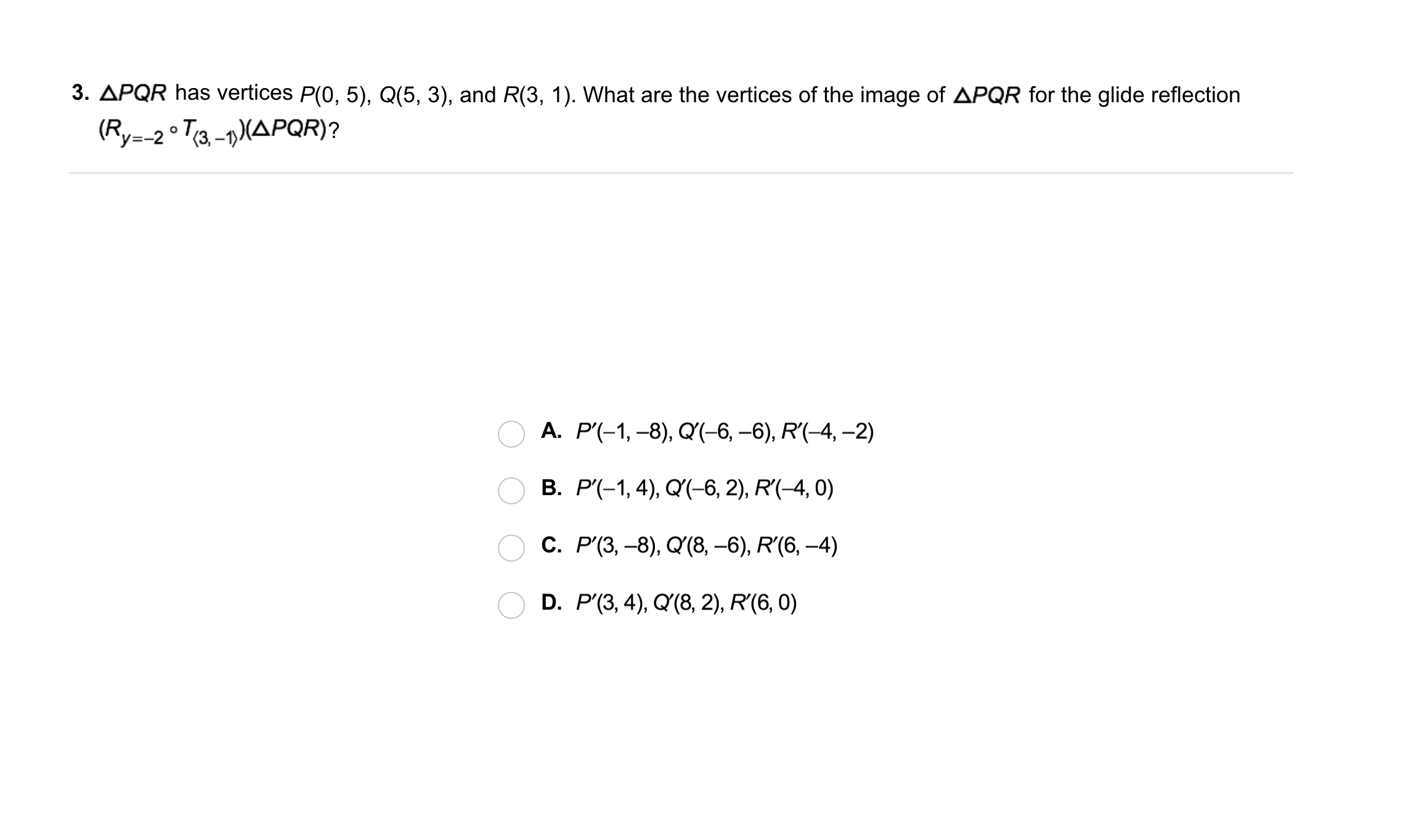12
12
10 pts
A
B
C
D14
14
10 pts
Error Analysis: You reflect ∆DEF first across line m and then across line n. Your friend says you can get the same result by reflecting ∆DEF first across line n and then across line m. Explain your friend's error.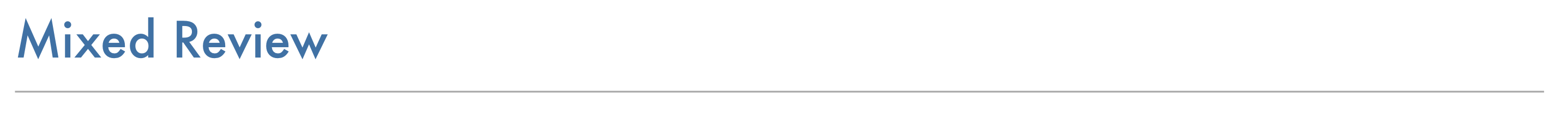15
10 pts
Review Lesson 9-3: Draw ∆ABC in black and its image for the rotation described in blue or green. Label all vertices.

16
10 pts
Review Lesson 9-3: Draw ∆ABC in black and its image for the rotation described in blue or green. Label all vertices.

17
10 pts
Review Lesson 5-5: Identify the two statements that contradict each other.
• △ABC is a right triangle.
• △ABC is equiangular.
• △ABC is isosceles.
• Statements that contradict each other
18
10 pts
Review Lesson 5-5: Identify the two statements that contradict each other.
• In right △ABC, m∠B = 90.
• In right △ABC, m∠A = 80.
• In right △ABC, m∠C = 90.
• Statements that contradict each other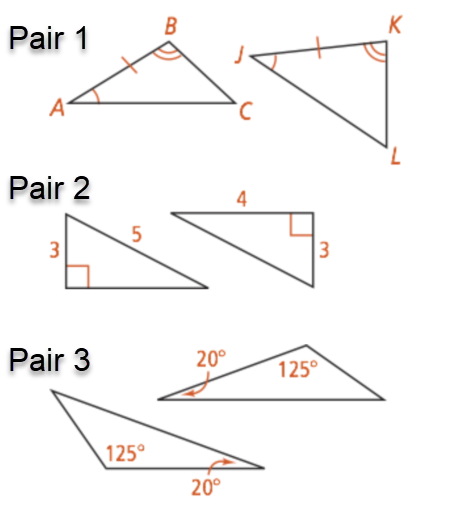19
19
10 pts
Review Lesson 4-2: Determine whether the triangles in each pair must be congruent. If so, name the postulate of theorem that justifies your answer.
• Pair 1
• Pair 2
• Pair 3
• Not necessarily congruent
• SSS
• ASA20
10 pts
Vocabulary Review: Match the pairs of corresponding sides.
• segment JQ
• segment KQ
• segment J'K'
• segment J'Q'
• segment JK
• segment K'Q'
21
10 pts
Vocabulary Review: Complete each sentence.
• reflection
• ∠K'
• translation
• ∠J
• ∠Q
• rotation
• ∠J'
• dilation
• The image of ∠J is __?__.
• The transformation △JKQ → △J'K'Q' is a __?__.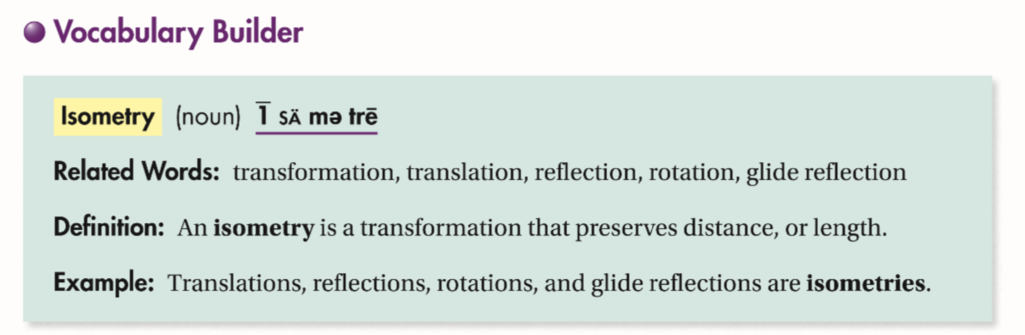22
10 pts
Use Your Vocabulary: Complete each sentence with image or preimage.
• image
• preimage
• In a transformation, the original figure is the __?__.
• The resulting figure is the __?__.
23
10 pts
Use Your Vocabulary: Which type of transformation maps each (x, y) to (x - 8, y + 2)?
translation
reflection
rotation
glide reflection
dilation
24
10 pts
Reflection: Math Success